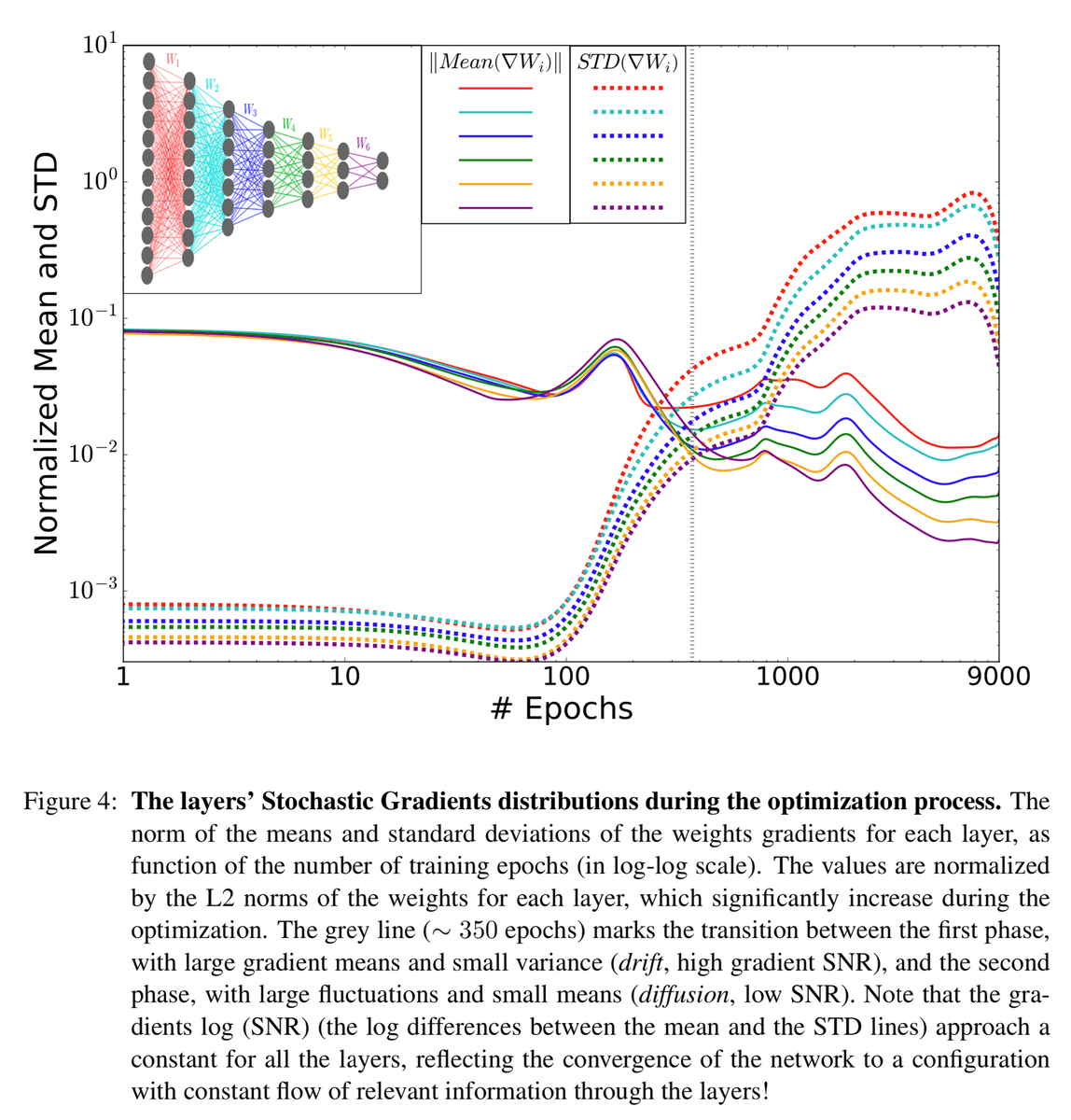# Deep Neural Network (DNN)

Researchers' study | The MIT research trio of Tomaso Poggio, Andrzej Banburski, and Quianli Liao - Center for Brains, Minds, and Machines) compared deep and shallow networks in which both used identical sets of procedures such as pooling, convolution, linear combinations, a fixed nonlinear function of one variable, and dot products. Why do deep networks have great approximation powers, and tend to achieve better results than shallow networks given they are both universal approximators?

The scientists observed that with convolutional deep neural networks with hierarchical locality, this exponential cost vanishes and becomes more linear again. Then they demonstrated that dimensionality can be avoided for deep networks of the convolutional type for certain types of compositional functions. The implications are that for problems with hierarchical locality, such as image classification, deep networks are exponentially more powerful than shallow networks. ...

The scientists observed that with convolutional deep neural networks with hierarchical locality, this exponential cost vanishes and becomes more linear again. Then they demonstrated that dimensionality can be avoided for deep networks of the convolutional type for certain types of compositional functions. The implications are that for problems with hierarchical locality, such as image classification, deep networks are exponentially more powerful than shallow networks.

“In approximation theory, both shallow and deep networks are known to approximate any continuous functions at an exponential cost,” the researchers wrote. “However, we proved that for certain types of compositional functions, deep networks of the convolutional type (even without weight sharing) can avoid the curse of dimensionality.”

The team then set out to explain why deep networks, which tend to be over-parameterized, perform well on out-of-sample data. The researchers demonstrated that for classification problems, given a standard deep network, trained with gradient descent algorithms, it is the direction in the parameter space that matters, rather than the norms or the size of the weights.

The implications are that the dynamics of gradient descent on deep networks are equivalent to those with explicit constraints on both the norm and size of the parameters–the gradient descent converges to the max-margin solution. The team discovered a similarity known to linear models in which vector machines converge to the pseudoinverse solution which aims to minimize the number of solutions.

In effect, the team posit that the act of training deep networks serves to provide implicit regularization and norm control. The scientists attribute the ability for deep networks to generalize, sans explicit capacity controls of a regularization term or constraint on the norm of the weights, to the mathematical computation that shows the unit vector (computed from the solution of gradient descent) remains the same, whether or not the constraint is enforced during gradient descent. In other words, deep networks select minimum norm solutions, hence the gradient flow of deep networks with an exponential-type loss locally minimizes the expected error. A New AI Study May Explain Why Deep Learning Works MIT researchers’ new theory illuminates machine learning’s black box.| Cami Rosso - Psychology Today ..PNAS (Proceedings of the National Academy of Sciences of the United States of America) | T. Poggio, A. Banburski, and Q. Liao

## Neural Networks - Hugo Larochelle

### Opening the Black Box